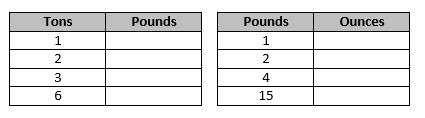# Unit Conversions

## Objective

Express customary weight measurements in terms of a smaller unit, recording measurement equivalents in a two-column table. Solve one-step word problems that require customary weight unit conversion.

## Materials and Resources

• Weights  — 1 one-pound, 16 one-ounce (Optional: 1 per teacher/student or group of students)

• Balance scale

## Common Core Standards

### Core Standards

?

• 4.MD.A.1 — Know relative sizes of measurement units within one system of units including km, m, cm; kg, g; lb, oz.; l, ml; hr, min, sec. Within a single system of measurement, express measurements in a larger unit in terms of a smaller unit. Record measurement equivalents in a two column table. For example, know that 1 ft is 12 times as long as 1 in. Express the length of a 4 ft snake as 48 in. Generate a conversion table for feet and inches listing the number pairs (1, 12), (2, 24), (3, 36), …

• 4.MD.A.2 — Use the four operations to solve word problems involving distances, intervals of time, liquid volumes, masses of objects, and money, including problems involving simple fractions or decimals, and problems that require expressing measurements given in a larger unit in terms of a smaller unit. Represent measurement quantities using diagrams such as number line diagrams that feature a measurement scale.

?

• 3.MD.A.2

• 4.OA.A.1

## Criteria for Success

?

1. Establish benchmarks for the customary unit of a ton, pound, and ounce.
2. Understand that a ton is 2,000 times heavier than a pound and a pound is 16 times heavier than a ton.
3. Use these relationships to convert measurements from a larger customary weight unit to a smaller unit (MP.7, MP.8).
4. Use these relationships to convert measurements from mixed customary weight units to a smaller unit (MP.7, MP.8).
5. Solve one-step word problems that require customary weight unit conversions (MP.4).

## Tips for Teachers

?

• It is unclear whether converting from tons to pounds is expected in Grade 4. It is not explicitly mentioned in the CCSS, the Progressions, or standardized test items from the larger testing sources (SBAC, PARCC, MCAS, and NY State Math Test). However, because the unit exists on the Massachusetts Comprehensive Assessment System Grade 5 Mathematics Reference Sheet and is therefore assumed to be a conversion students need to be familiar with, it is included in this lesson. It is at your discretion to choose to cut it.
• As mentioned in Lesson 2, the term “mass” is used through Lesson 2 in reference to metric mass measurement but the term “weight” is used throughout Lesson 6 in reference to customary weight measurement. Enforcing the correct usage with students isn’t necessary but, it could be discussed if a student raises the issue.

#### Fishtank Plus

• Problem Set
• Student Handout Editor
• Vocabulary Package

?

### Problem 1

Use a balance scale and one-pound and one-ounce weights to answer the following question.

What do you notice about the relationship between the weight of a pound and the weight of an ounce?

### Problem 2

Fill in the following conversion tables.### Problem 3

A birth weight between 5 pounds 8 ounces and 8 pounds 13 ounces is considered normal for a full-term newborn. Louis’s daughter weighs 145 ounces. Is Louis’s daughter’s weight considered normal?

### Problem 4

Robyn and Danny are truck drivers. They have to haul the following pallets to the same location.

 Pallet Weight A 4,000 lb B 4 tons 1,000 lb C 1 ton 1,625 lb D 1,000 lb E 3 tons F 2 tons 375 lb

Robyn and Danny want to each haul the same amount of weight. They can’t break the pallets apart into smaller packages. Which pallets should they each take? How much weight will they be hauling?

## Discussion of Problem Set

?

• Did anyone determine how many ounces are in a ton in #3? How did you figure it out?
• How many additional one-ounce weights are needed to balance Julius’s three-pound weight in #4? How did you figure that out?
• In #5, I think each bottle of glue weighs 2 ounces, since 1 lb. 2 oz. is the same as 12 ounces. Do you agree or disagree? Why?
• What strategy did you use to solve #6? Which strategy is most efficient?
• When measuring weight, which customary unit could I use? Which metric unit could I use?

?

### Problem 1

Solve.

a. 9 pounds = __________ ounces

b. 13 pounds 5 ounces = __________ ounces

### Problem 2

Sasha’s backpack weighs 15 pounds 9 ounces. Amanda’s backpack weighs 183 ounces. Stefan’s backpack weighs 272 ounces. Order the weight of each backpack from greatest to least.

?